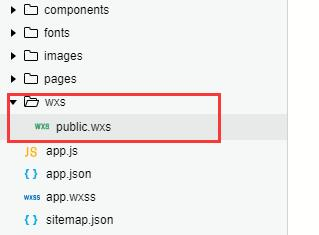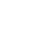# 微信小程序wxs时间戳格式化多功能转换方法```  myDate: function (value, type = 0){
var time = getDate(value);
var year = time.getFullYear();
var month = time.getMonth() + 1;
var date = time.getDate();
var hour = time.getHours();
var minute = time.getMinutes();
var second = time.getSeconds();
month = month < 10 ? "0" + month : month;
date = date < 10 ? "0" + date : date;
hour = hour < 10 ? "0" + hour : hour;
minute = minute < 10 ? "0" + minute : minute;
second = second < 10 ? "0" + second : second;
var arr = [
year + "-" + month + "-" + date,
year + "-" + month + "-" + date + " " + hour + ":" + minute + ":" + second,
year + "年" + month + "月" + date,
year + "年" + month + "月" + date + " " + hour + ":" + minute + ":" + second,
hour + ":" + minute + ":" + second
]
return arr[type];
}
``````var public={
myDate:function(){
//这里放置时间格式化代码
}
}
module.exports=public```

```<wxs module="public" src="../../wxs/public.wxs"/>
<view>{{public.myDate(1585472088000)}}</view>```3530